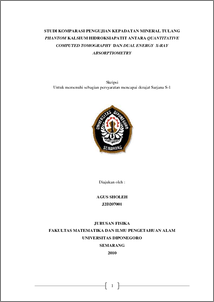# STUDI KOMPARASI PENGUJIAN KEPADATAN MINERAL TULANG PHANTOM KALSIUM HIDROKSIAPATIT ANTARA QUANTITATIVE COMPUTED TOMOGRAPHY DAN DUAL ENERGY X-RAY ABSORPTIOMETRY

Sholeh, Agus (2010) STUDI KOMPARASI PENGUJIAN KEPADATAN MINERAL TULANG PHANTOM KALSIUM HIDROKSIAPATIT ANTARA QUANTITATIVE COMPUTED TOMOGRAPHY DAN DUAL ENERGY X-RAY ABSORPTIOMETRY. Undergraduate thesis, Department of Physics, Diponegoro University.Preview
PDF
43Kb

## Abstract

Has been realized, making calcium hydroxyapatite phantom (phantom CaHA) in agar gel matrix with CaHA density () 80 mg/cm3, 100 mg/cm3, 120 mg/cm3, 140 mg/cm3,160 mg/cm3, 180 mg/cm3, and 200 mg/cm3). Phantom CaHA density tested with QCT and Dexa.. The both test results of bone mineral density compared to each other. In the QCT measurement data obtained by volumetric density of bone mineral,(QCT), in units of mass per cm3 and the linear attenuation coefficient of each phantom CaHA. Dexa measurement data obtained by area density of bone mineral, M(Dexa), in units of mass per cm2 and the composition of fatty tissue in units of percent (%). Area density (M) is the product of volumetric density () with phantom thickness. Data (QCT) multiplied by the thickness of phantom to obtain the area density M(QCT). The result of this calculation is compared with M(Dexa). While data M(Dexa) divided by phantom thickness to obtain the volumetric density (Dexa). The result of this calculation is compared with (QCT). Results showed a comparative value test density area between QCT and Dexa is M(QCT) = 45.95 +1.07 M(Dexa) and comparisons of voumetric density values test between Dexa and QCT is (Dexa) = -12.17 +0 , 92 (QCT) with r = 0.996 and R = 0.992.

Item Type: Thesis (Undergraduate) calcium hydroxyapatite phantom (phantom CaHA), QCT, Dexa, the linear attenuation coefficient Q Science > QC Physics Faculty of Science and Mathematics > Department of Physics 24233 Ms. Wien Admin 29 Nov 2010 11:22 29 Nov 2010 11:22

Repository Staff Only: item control page